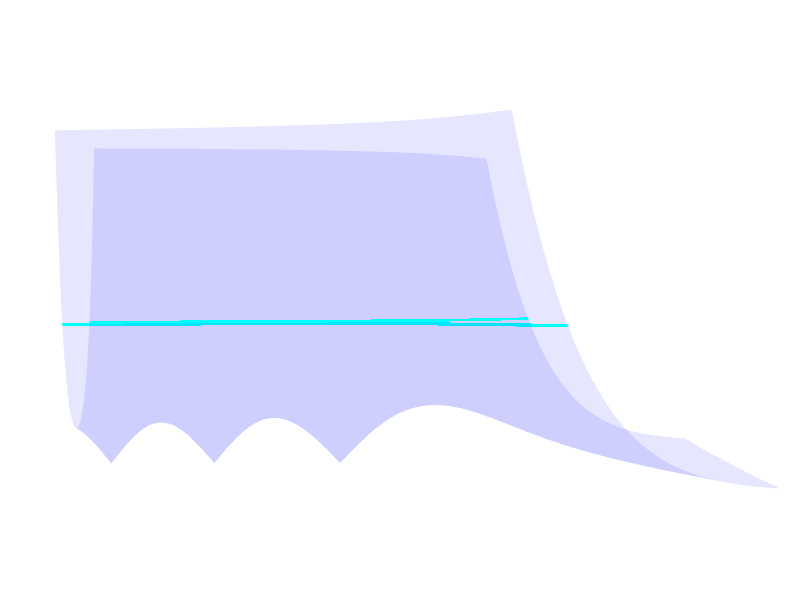# DLMF Model Viewer

## Three.js

The aim of the project is to create a lightweight 3D library with a very low level of complexity

• open-source Javascript 3D library
• simplifies usage of WebGL

## DLMF

### Digital Library of Mathematical Functions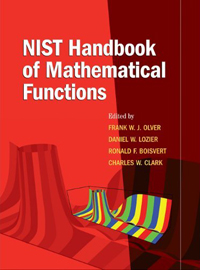Electronic version of NIST Handbook of Mathematical Functions Provides information, visualizations of complex equations

A computer program that is used primarily to calculate rendering effects on graphics hardware with a high degree of flexibility.

## Graphics Pipeline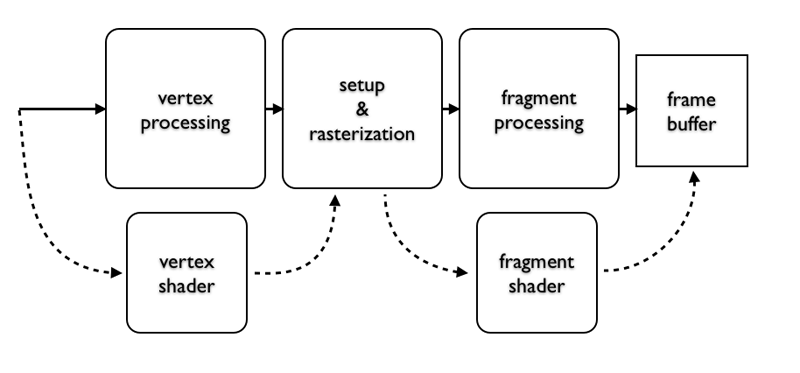## My Project

• Explore model visualization with WebGL
• Interesting coloring
• Arbitrary cutting plane

## Creating an Arbitrary Cutting Plane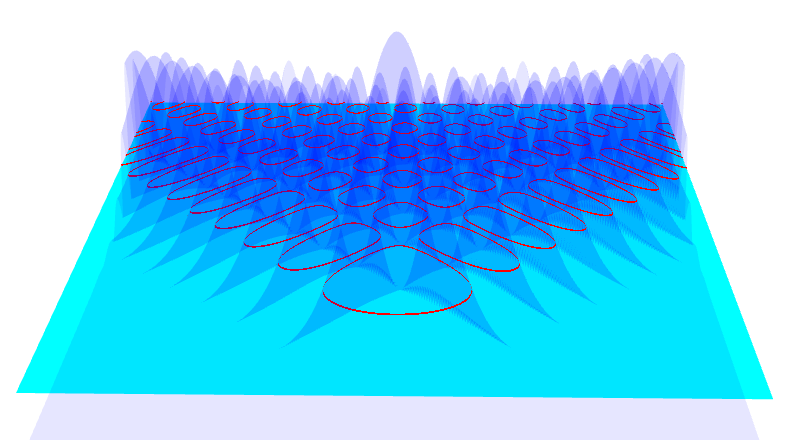### Three.js Plane Definition

• `THREE.PlaneGeometry`
• Visual plane → width and depth
• Appears as a square in 3D space
• Four vertices

### Problems

• Need to check plane against points on figure
• 3D figure vertices are not dense

### Work-arounds

• Check clipping using 2 planes
• Use dot product of vertex and plane for both psuedo-planes

## Mathematic and Programmatic Concepts

### Javascript

Represent plane as normal vector and offset

ax + by + cz + d = 0

Use function to create 2 planes

``````
backPlaneEquation = getPlaneEquation(clippingPlane, -planeOffset);
frontPlaneEquation = getPlaneEquation(clippingPlane, planeOffset);
``````

Which gets you something that looks like this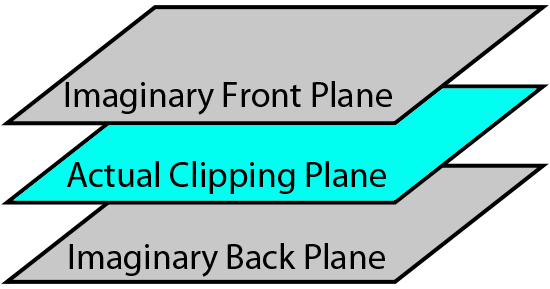### GLSL

``````
varying vec4 vertexPosition;

void main() {
vertexPosition = vec4(position, 1.0);
...
}
``````

Fragment Shader: Check if vertex is in between planes

``````
if (dot(frontPlane, vertexPosition) >= 0.0 && dot(backPlane, vertexPosition) < 0.0) {
// Vertex is between planes, display it differently
} else {
// Vertex is not between planes, display it normally
}
``````

### End Result